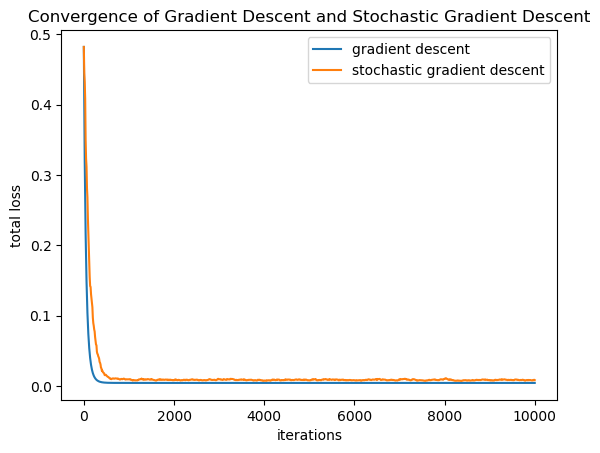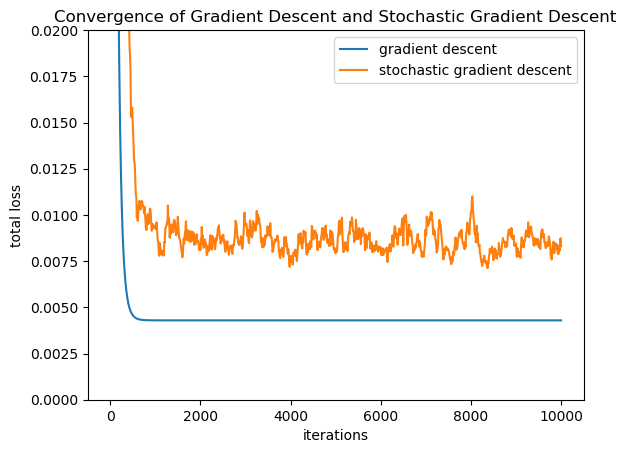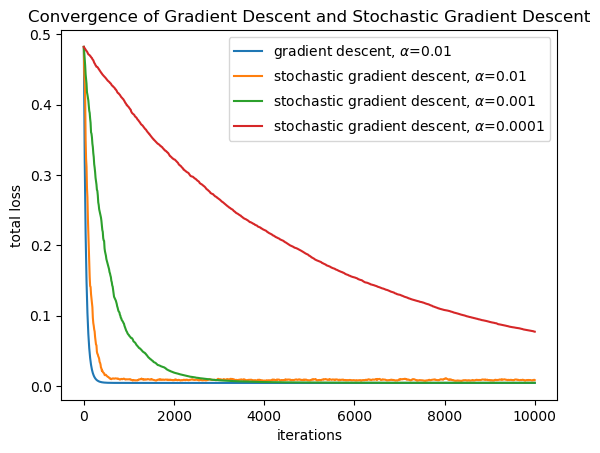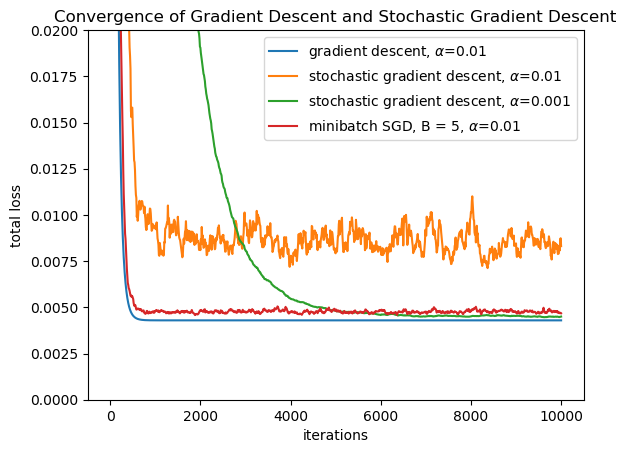In :
using PyPlot
using LinearAlgebra
using Statistics
using Random

Random.seed!(1234567);

┌ Warning: PyPlot is using tkagg backend, which is known to cause crashes on MacOS (#410); use the MPLBACKEND environment variable to request a different backend.
└ @ PyPlot /Users/cdesa/.julia/packages/PyPlot/4wzW1/src/init.jl:192


### GD vs SGD example: linear regression¶

Loss function is $$f_i(w) = \frac{1}{2} (w^T x_i - y_i)^2.$$ We'll sample a random dataset over $d$ dimensions in which $$x_i \sim \mathcal{N}(0, I)$$ and for some constant unit vector $u$, $$y_i = u^T x_i + \mathcal{N}(0,0.1^2).$$

In :
struct LinRegDataset
Xs :: Array{Array{Float64,1},1}
Ys :: Array{Float64,1}
end

function generate_synthetic_dataset(d::Int64, n::Int64)
Random.seed!(8675309);

# generate random unit vector
u = randn(d); u /= norm(u);

Xs = [randn(d) for i = 1:n];
Ys = [dot(u, Xs[i]) + 0.1 * randn() for i = 1:n];

return LinRegDataset(Xs, Ys);
end

d = 100;
n = 1000;

TD = generate_synthetic_dataset(d, n);

In :
function total_loss(TD :: LinRegDataset, w :: Array{Float64,1})
return mean([(dot(w, TD.Xs[i]) - TD.Ys[i])^2 / 2 for i = 1:n]);
end

Out:
total_loss (generic function with 1 method)
In :
total_loss(TD, zeros(d))

Out:
0.48214926002770636
In :
function grad_fi(TD :: LinRegDataset, i::Int64, w :: Array{Float64,1})
return TD.Xs[i] * (dot(w, TD.Xs[i]) - TD.Ys[i]);
end

function total_grad(TD :: LinRegDataset, w :: Array{Float64,1})
n = length(TD.Xs);
return mean([grad_fi(TD, i, w) for i = 1:n]);
end

Out:
total_grad (generic function with 1 method)
In :
function gradient_descent(TD :: LinRegDataset, alpha :: Float64, num_iters :: Int64, loss_interval :: Int64)
n = length(TD.Xs);
d = length(TD.Xs);
# initialize the parameters to zero
w = zeros(d);
# vector to store loss after t iterations
measurement_times = Float64;
loss_at_t = Float64[total_loss(TD, w)];
# run iteratively
for t = 1:num_iters
w = w - alpha * total_grad(TD, w);
if t % loss_interval == 0
push!(measurement_times, t);
push!(loss_at_t, total_loss(TD, w));
end
end
return (measurement_times, loss_at_t)
end

function stochastic_gradient_descent(TD :: LinRegDataset, alpha :: Float64, num_iters :: Int64, loss_interval :: Int64)
n = length(TD.Xs);
d = length(TD.Xs);
# initialize the parameters to zero
w = zeros(d);
# vector to store loss after t iterations
measurement_times = Float64;
loss_at_t = Float64[total_loss(TD, w)];
# run iteratively
for t = 1:num_iters
i = rand(1:n);
w = w - alpha * grad_fi(TD, i, w);
if t % loss_interval == 0
push!(measurement_times, t);
push!(loss_at_t, total_loss(TD, w));
end
end
return (measurement_times, loss_at_t)
end

Out:
stochastic_gradient_descent (generic function with 1 method)
In :
alpha = 0.01;
num_iters = 10000;
(gd_t, gd_loss) = gradient_descent(TD, alpha, num_iters, 10);
(sgd_t, sgd_loss) = stochastic_gradient_descent(TD, alpha, num_iters, 10);

# also, benchmark the time it takes to run

  4.988261 seconds (20.05 M allocations: 16.782 GiB, 42.66% gc time)
0.004151 seconds (30.02 k allocations: 25.652 MiB)

In :
plot(gd_t, gd_loss; label="gradient descent");
legend();
xlabel("iterations");
ylabel("total loss");In :
plot(gd_t, gd_loss; label="gradient descent");
legend();
xlabel("iterations");
ylabel("total loss");
ylim([0.0,0.02]);Notice that SGD with a constant step size did not converge asymptotically to as low of a loss that gradient descent did. Instead, it converged down to some level, and then the loss stopped decreasing and the value of the loss is noisy. This is sometimes called converging to a noise ball.

Often, converging to a noise ball is fine: we don't always need the exact solution to the optimization problem.

Question: Why might it be fine to get an approximate solution to an optimization problem for training?

.

.

.

.

Still, it is good to be able to solve optimization problems to arbitrary accuracy if we want to. We can do this with stochastic gradient descent by decreasing the step size. Even using a smaller constant step size will work.

In :
alpha2 = 0.001;
(sgd_t2, sgd_loss2) = stochastic_gradient_descent(TD, alpha2, num_iters, 10);
alpha3 = 0.0001;
(sgd_t3, sgd_loss3) = stochastic_gradient_descent(TD, alpha3, num_iters, 10);

In :
plot(gd_t, gd_loss; label="gradient descent, \$\\alpha\$=$alpha"); plot(sgd_t, sgd_loss; label="stochastic gradient descent, \$\\alpha\$=$alpha");
plot(sgd_t2, sgd_loss2; label="stochastic gradient descent, \$\\alpha\$=$alpha2"); plot(sgd_t3, sgd_loss3; label="stochastic gradient descent, \$\\alpha\$=$alpha3");
legend();
xlabel("iterations");
ylabel("total loss");

#ylim([0.0,0.02]);Question: What trade-off is illustrated in this figure?

Another alternative: rather than making the step size smaller, make the gradient estimates more accurate by using a sample size larger than $1$ (remember Hoeffding's inequality: as the sample size gets larger the error of the estimates becomes smaller). This is called minibatch SGD.

In :
function minibatch_stochastic_gradient_descent(TD :: LinRegDataset, alpha :: Float64, batch_size :: Int64, num_iters :: Int64, loss_interval :: Int64)
n = length(TD.Xs);
d = length(TD.Xs);
# initialize the parameters to zero
w = zeros(d);
# vector to store loss after t iterations
measurement_times = Float64;
loss_at_t = Float64[total_loss(TD, w)];
# run iteratively
for t = 1:num_iters
is = rand(1:n, batch_size);
w = w - alpha * mean([grad_fi(TD, i, w) for i in is]);
if t % loss_interval == 0
push!(measurement_times, t);
push!(loss_at_t, total_loss(TD, w));
end
end
return (measurement_times, loss_at_t)
end

Out:
minibatch_stochastic_gradient_descent (generic function with 1 method)
In :
batch_size = 5;

(sgd_t3, sgd_loss3) = minibatch_stochastic_gradient_descent(TD, alpha, batch_size, num_iters, 10);

# also, benchmark the time it takes to run, compared with plain SGD
@time minibatch_stochastic_gradient_descent(TD, alpha, batch_size, num_iters, num_iters);

  0.005356 seconds (30.02 k allocations: 25.652 MiB, 33.50% gc time)
0.016987 seconds (160.02 k allocations: 105.608 MiB, 16.27% gc time)

In :
plot(gd_t, gd_loss; label="gradient descent, \$\\alpha\$=$alpha"); plot(sgd_t, sgd_loss; label="stochastic gradient descent, \$\\alpha\$=$alpha");
plot(sgd_t2, sgd_loss2; label="stochastic gradient descent, \$\\alpha\$=$alpha2"); plot(sgd_t3, sgd_loss3; label="minibatch SGD, B =$batch_size, \$\\alpha\$=\$alpha");
legend();